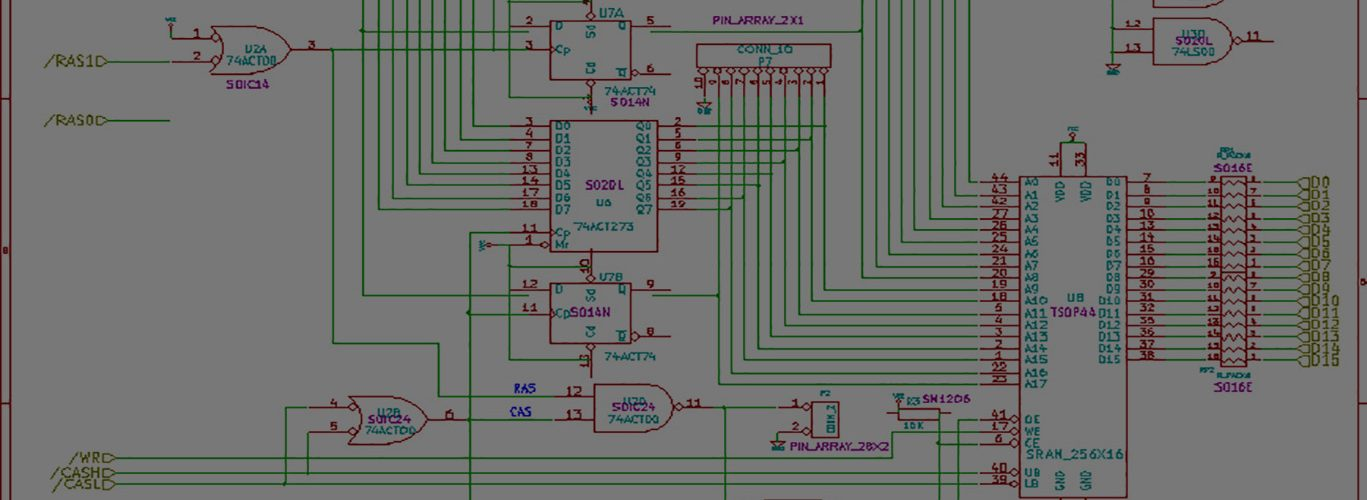# on floppy adpll, this time, my solution

This isn’t by any stretch finished, but it does do what I’ve expected it to do.

It responds both to differences in phase (counter is set to 0), and differences in frequency (period is adjusted in the direction of the frequency error)

I did this at 3am last night, so there could be a couple bugs in there.

With all this being said, I’m not entirely sure that PLLs are actually required for good reading of amiga floppy disks. My regular “static interval” solution works about 95%-98% of the time. I’m going to come up with a list of problem disks and see if this solution works better/worse/otherwise.

I’ve used a read data window of 1us, which starts being centered on 2us, and is automatically adjusted as data comes in. This produces windows around 2us, 4us, 6us, 8us. I output the overall error which is the deviation from the center of the window as each pulse is received. I’d like to graph this error, but it doesn’t look like Xilinx’s iSim will export a particular output as CSV or whatever.

module floppy_pll(
input clk,
input floppy_data,
input reset,
output reg window_open,
output reg [7:0] data,
output reg strobe,
output reg [7:0] error
);

// window is 1us wide
// starts at .5us before counter rollover
// ends .5us after counter rollover
// ideally, edges should be arriving right when the counter rolls over

reg [7:0] period = 100;
reg [10:0] counter = 0;

reg IN_D1, IN_D2;
wire out_negedge = ~IN_D1 & IN_D2;

always@(posedge clk or posedge out_negedge) begin

if (reset) period <= 100;

if (clk) begin

counter <= counter + 1;
if (counter == period) counter <= 0;

if ( (counter > (period-25)) || (counter < 25) ) window_open <= 1;
else window_open <= 0;

end

if (out_negedge) begin

// if counter == 0 and we see out_negedge be positive, then we are perfectly aligned
// so we dont need to adjust anything
if (counter != 0) begin

if (window_open) begin

counter <= 0; // align counter to the phase of the incoming signal

if (counter < 25) begin

//we rolled over before pulse was seen, so make period larger
//error values will be over 128
period <= period + 1;
error <= (128 + counter);

end

if (counter > (period-25)) begin

//we haven't rolled when we saw the pulse, so make period shorter
//error values will be less than 128

period <= period - 1;
error <= 128 - (period-counter);

end

end

end

end

// edge detection flops for data in direct from floppy
IN_D1 <= floppy_data;
IN_D2 <= IN_D1;
end

endmodule#### keith

Amateur Electronics Design Engineer and Hacker